Question

# programming in C a study guide question. how can I access an element of a dynamically...

programming in C a study guide question.

how can I access an element of a dynamically allocated array without using [] operator

Given ar2 is defined as int** ar2, access ar2[i][j] without using the [] operator.Editable code

for (int i = 0; i < 4; i++) {

for (int j = 0; j < 4; j++) {

if(i==2 && j==1)

printf("%d", *(*(p + i)+j));

}

}

#### Earn Coins

Coins can be redeemed for fabulous gifts.

Similar Homework Help Questions
• ### Using C programming

Using C, create a data file with the first number being an integer. The value of that integer will be the number of further integers which follow it in the file. Write the code to read the first number into the integer variable how_many.Please help me with the file :((This comes from this question:Write the code to dynamically allocate ONE integer variable using calloc (contiguous allocation) or malloc (memory allocation) and have it pointed to by a pointer (of type int...

• ### Write the code to dynamically allocate ONE integer variable using calloc (contiguous allocation) or malloc (memory...

Write the code to dynamically allocate ONE integer variable using calloc (contiguous allocation) or malloc (memory allocation) and have it pointed to by a pointer (of type int * ) named ptr_1. Use ptr_1 to assign the number 7 to that dynamically allocated integer, and in another line use printf to output the contents of that dynamically allocated integer variable. Write the code to dynamically allocate an integer array of length 5 using calloc or malloc and have it pointed...

• ### C programming only please 5.2.3: Printing array elements with a for loop. Write a for loop...

C programming only please 5.2.3: Printing array elements with a for loop. Write a for loop to print all elements in courseGrades, following each element with a space (including the last). Print forwards, then backwards. End each loop with a newline. Ex: If courseGrades = {7, 9, 11, 10}, print: 7 9 11 10 10 11 9 7 Hint: Use two for loops. Second loop starts with i = NUM_VALS - 1. (Notes) Note: These activities may test code with...

• ### Programming Question 4: Operator Overloading [20 marks] The class SpecialArray represents an arra...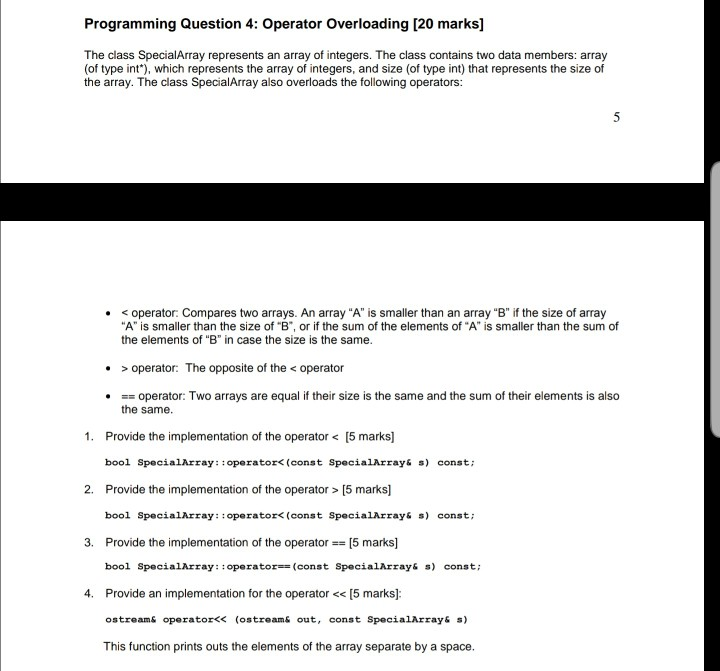C++ please Programming Question 4: Operator Overloading [20 marks] The class SpecialArray represents an array of integers. The class contains two data members: array (of type int"), which represents the array of integers, and size (of type int) that represents the size of the array. The class SpecialArray also overloads the following operators: operator: Compares two arrays. An array "A" is smallerhan an array "B" if the size of array "A" is smaller than the size of "B", or if...

• ### Create the program in C++ please. The new operator as outlined in 10.9 . Create a...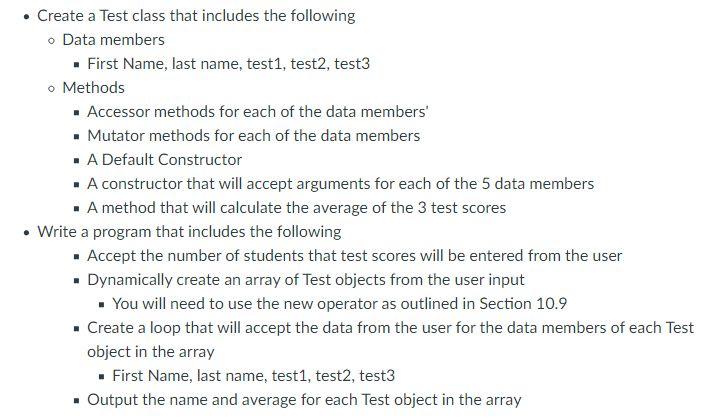Create the program in C++ please. The new operator as outlined in 10.9 . Create a Test class that includes the following Data members • First Name, last name, test1, test2, test3 o Methods • Accessor methods for each of the data members' • Mutator methods for each of the data members . A Default Constructor . A constructor that will accept arguments for each of the 5 data members • A method that will calculate the average of the...

• ### Hey everyone, I need help making a function with this directions with C++ Language. Can you...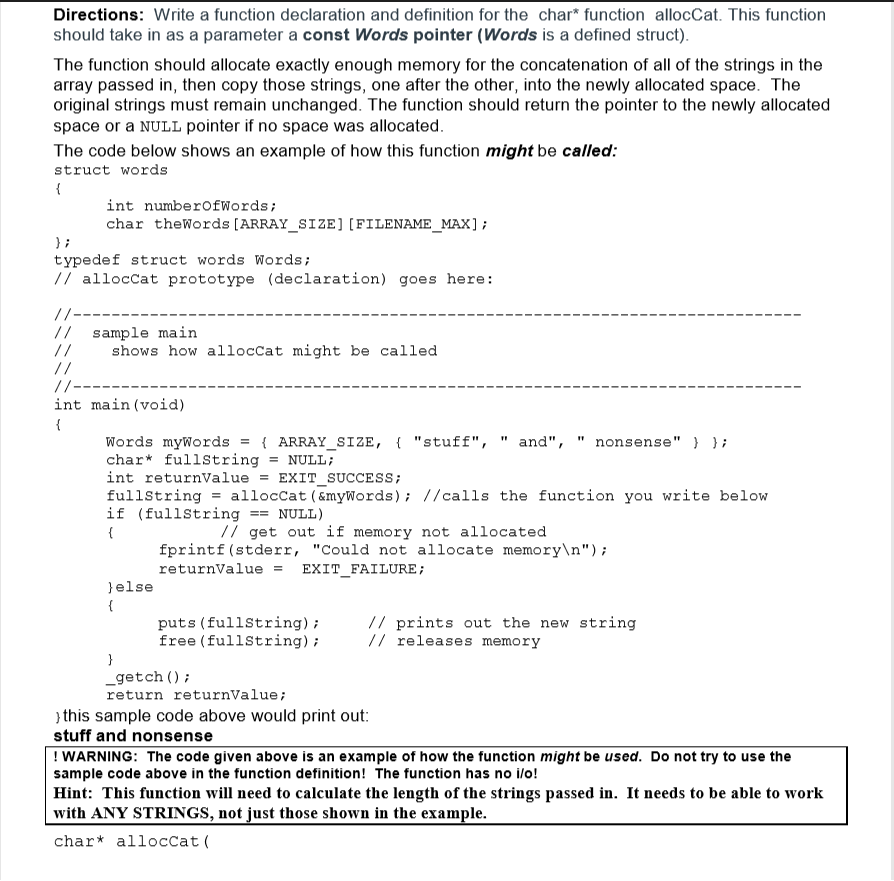Hey everyone, I need help making a function with this directions with C++ Language. Can you guys use code like printf and fscanf without iostream or fstream because i havent study that yet. Thanks. Directions: Write a function declaration and definition for the char* function allocCat. This function should take in as a parameter a const Words pointer (Words is a defined struct) The function should allocate exactly enough memory for the concatenation of all of the strings in the...

• ### C++ ONLY! Consider the following code and answer the questions in the table below. #include <iostream>...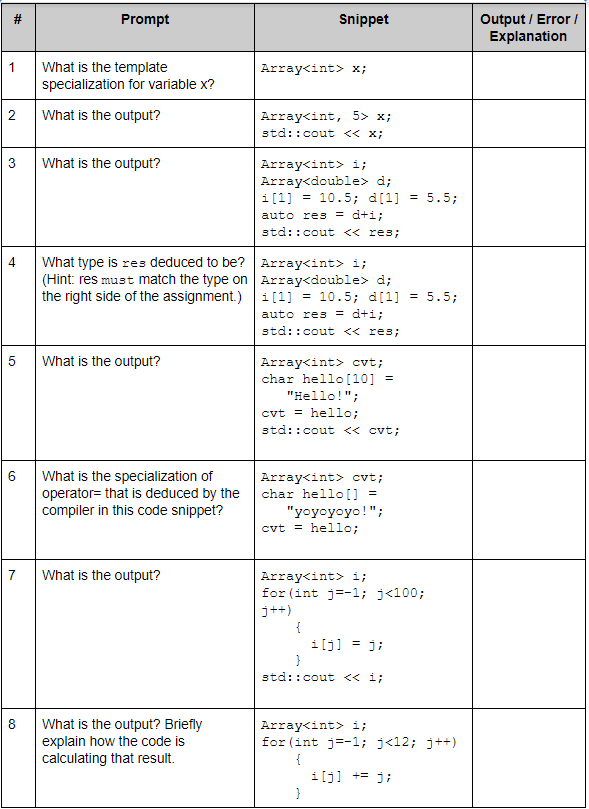C++ ONLY! Consider the following code and answer the questions in the table below. #include <iostream> template <typename T, int N=10> class Array { private:   T array[N+1]; public:   Array() {     for(int i=0; i<N+1; i++) array[i] = 0;   }      T& operator [] (int index) {     if (index>N || index < 0)       return array[N];     else       return array[index];   }   template <typename S>   Array<T,N>& operator= (S &other) {     for(int i=0; i<N+1; i++)       array[i] = other[i];         return *this;   } }; template <typename T, typename...

• ### basic c++ 2. AC++ program contains the following C++ code (assume an int occupies 2 bytes...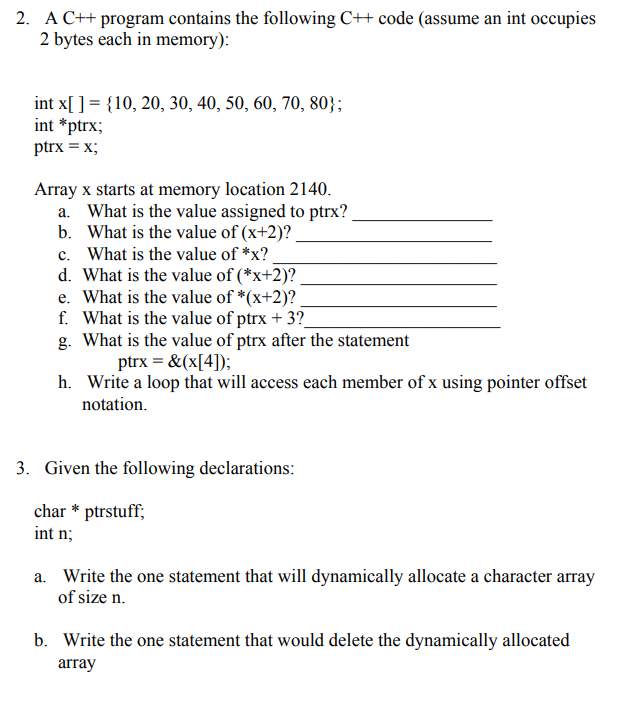basic c++ 2. AC++ program contains the following C++ code (assume an int occupies 2 bytes each in memory): int x[ ] = { 10, 20, 30, 40, 50, 60, 70, 80); int *ptrx ptrx = x; Array x starts at memory location 2140. a. What is the value assigned to ptrx? b. What is the value of (x+2)? c. What is the value of *x? d. What is the value of (*x+2)? e. What is the value of *(x+2)?...

• ### This is in java language. Please use simple java programming language. Each of the parts a....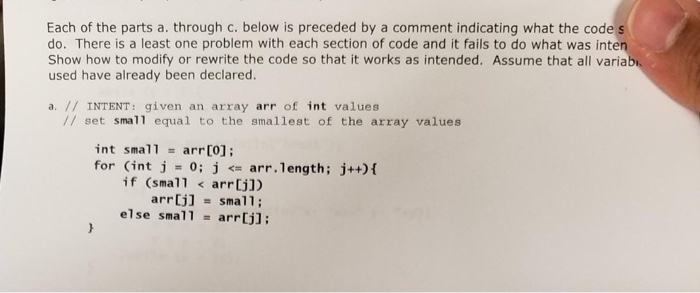This is in java language. Please use simple java programming language. Each of the parts a. through c. below is preceded by a comment indicating what the code do. There is a least one problem with each section of code and it fails to do what was inte Show how to modify or rewrite the code so that it works as intended. Assume that all varia used have already been declared. а. // INTENT : given an array arr of...

• ### I hope someone can explain this exercise to me. Thanks +++++++++++++ Programming Exercise Try to think...

I hope someone can explain this exercise to me. Thanks +++++++++++++ Programming Exercise Try to think about how to implement KWArrayList class. Please implement the following constructor and methods: public KWArrayList() public boolean add(E anEntry) public E get(int index) { public E set(int index, E newValue) public E remove(int index) private void reallocate() public int size() public int indexOf(Object item)       Study the code for ArrayList implementation (enclosed in the folder) and work on the following exercise Provide a constructor...